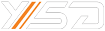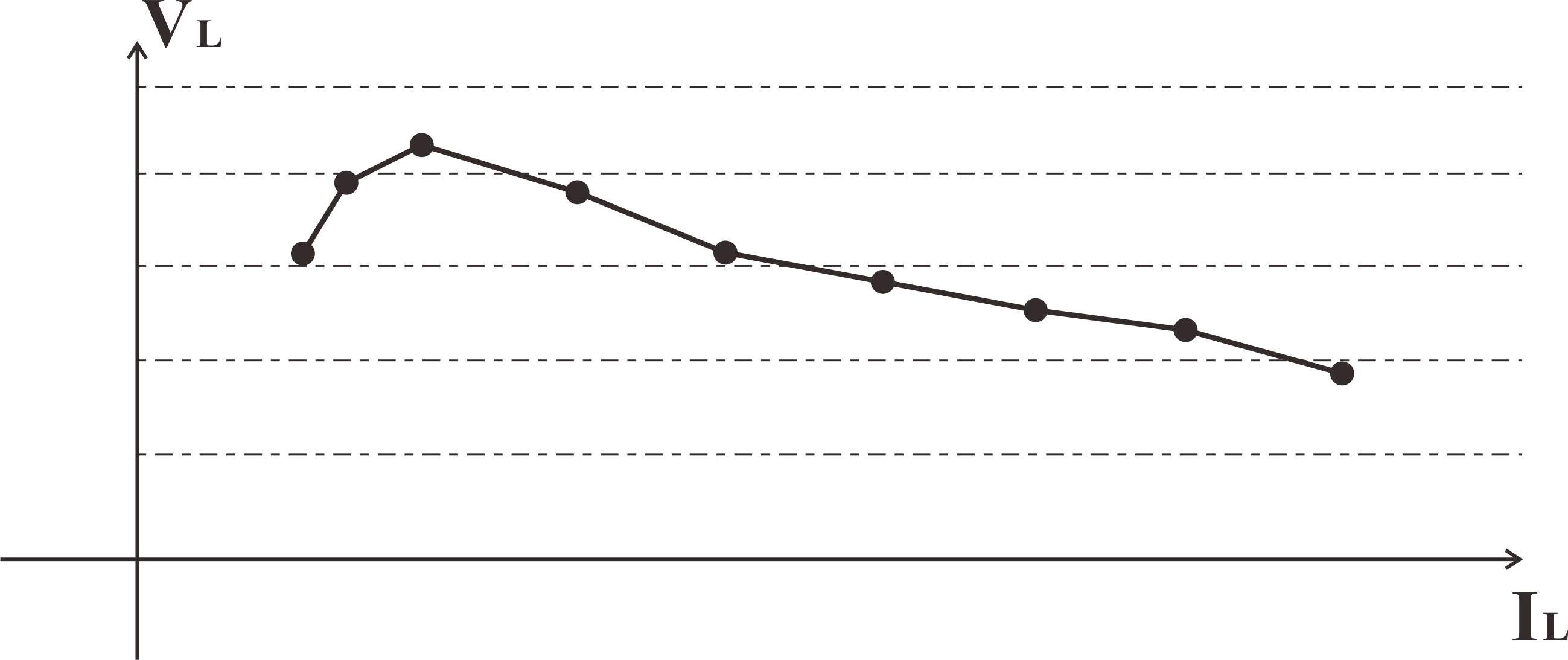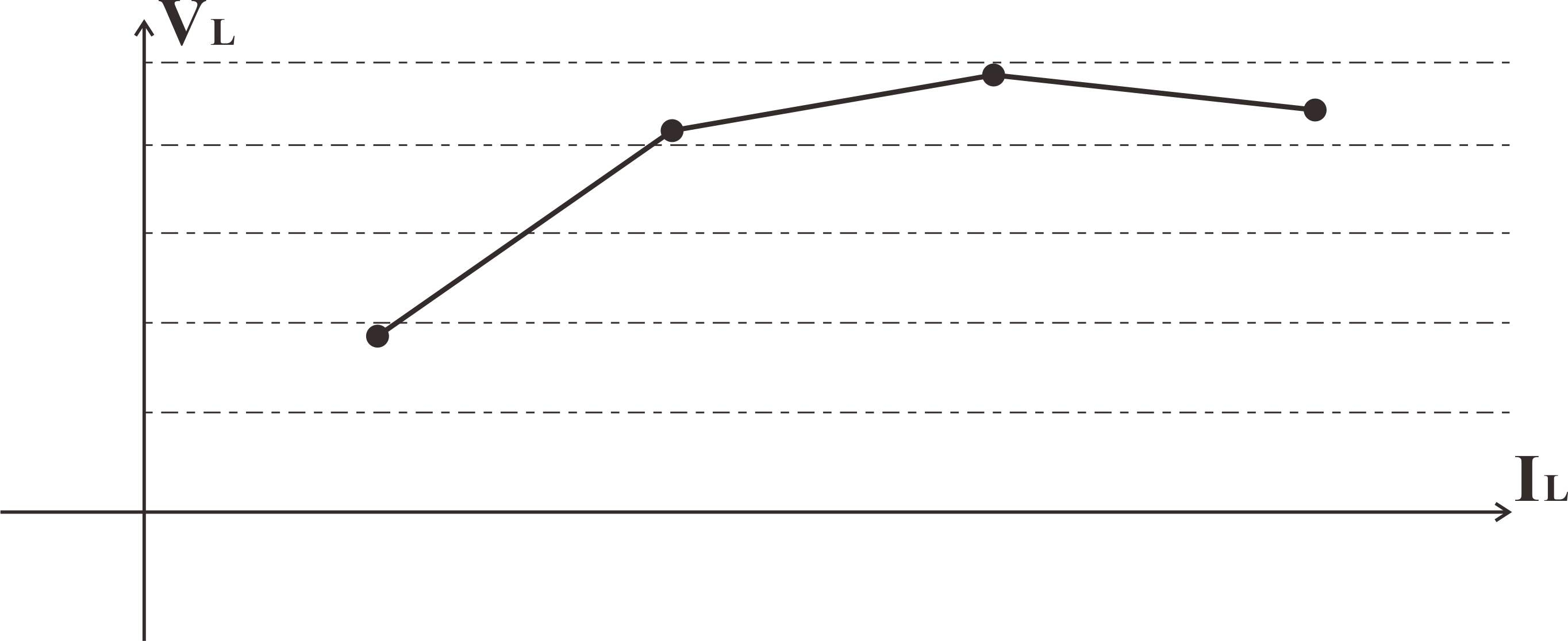#The difference between line regulation and load regulation and calculation formula
Your Position：Home>News>Industry-news>The difference between line regulation and load regulation and calculation formula

# The difference between line regulation and load regulation and calculation formula

There are actually two types of regulation that need to be paid attention to when choosing a power supply: load regulation and line regulation.

## Line regulation

Line regulation is the ability of the power supply to minimize the error of the constant output voltage when the input voltage changes. It is expressed as the percentage of the change in output voltage relative to the change in input line voltage. The power supply with strict line regulation capability can provide the best voltage in the entire working range.

When the input voltage source is unstable or unstable, line regulation is very important, which will cause a significant change in the output voltage. For most operations, the line regulation of the unregulated power supply is usually high, but it can be improved by using a voltage regulator. Low-voltage line regulation is always the first choice. Each pressure sensor that provides a high-level DC output signal should have a specified line adjustment rate. In fact, the line regulation rate of a well-regulated power supply is typically 0.02%, and the maximum should be 0.1%.

· Use line adjustment to calculate the change in output voltage

· With an example of a 1.8 V output LDO regulator whose input voltage varies from 3V to 4.2V, the extent of variations of the LDO output voltage by line regulation can be estimated as below:

· Input Voltage VariationΔVi4.2V-3V = 1.2V

· Based on the line regulation characteristicOutput Voltage VariationΔVois determined by the following formula

· Typ0.02/ V×1.2 V = 0.024   1.8 V×0.024= 0.432mv

· Max0.10%/ V × 1.2 V = 0.12%   1.8 V × 0.12% = 2.16 mv

· Thereforeinput voltage difference between 3V and 4.2V, the 1.8V output changes by typically 0.432 mV / maximum 2.16mV. The output voltage may be affected both positively and negatively.Load regulation is the ability of the power supply to maintain a constant output voltage (or current) under extremely light loads and loads close to the maximum current,One of the most important specifications of DC and AC power supplies is load regulation. Good load regulation will help ensure that the power supply can provide the voltage required by the circuit or system.

The following formula can get the load regulation rate:

· RTH is the output resistance of the supply,

· IFL the full load current (at the minimum resistance)

· RL(min) the minimum load resistance

Load adjustment rate is the standard to measure the quality of power supply.Good power output with small voltage drop at load.The smaller the load regulation rate, the more stable and reliable the power supply. If the load regulation rate of the power supply is poor, or when the load is suddenly removed, the output voltage will drop as the load increases or surges.## What are the detection methods for linear and load adjustment rates?

Linear adjustment rate: the output is fully loaded, the output voltage is measured within the full range of the input voltage, the oscilloscope and multimeter are observed, the maximum and minimum values of the output voltage when the full range of the input voltage changes are recorded, and the linear adjustment rate is obtained by using the above formula.

Load adjustment rate: the input is rated voltage, and the load is switched repeatedly under the two output conditions of no-load and full-load respectively.Observe the oscilloscope and multimeter, measure the amplitude and waveform of the output voltage, write down the maximum and minimum output voltage in the switching process, and obtain the load adjustment rate by using the above formula.Well-regulated power can ensure the normal operation of circuit components, otherwise fluctuations may cause circuit operation failure or component damage. Compared with linear power supplies, switching power supplies have better adjustment capabilities due to circuit design.